Documentation

## Arithmetic Operations

### Note

These sections will help you understand what data type and scaling choices result in overflows or a loss of precision.

### Modulo Arithmetic

Binary math is based on modulo arithmetic. Modulo arithmetic uses only a finite set of numbers, wrapping the results of any calculations that fall outside the given set back into the set.

For example, the common everyday clock uses modulo 12 arithmetic. Numbers in this system can only be 1 through 12. Therefore, in the “clock” system, 9 plus 9 equals 6. This can be more easily visualized as a number circle: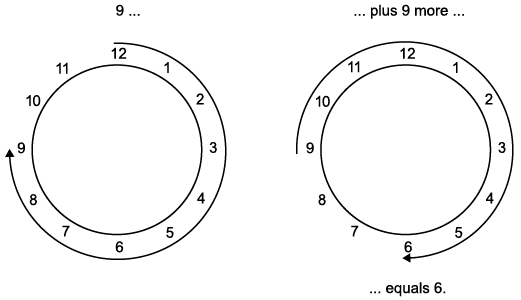Similarly, binary math can only use the numbers 0 and 1, and any arithmetic results that fall outside this range are wrapped “around the circle” to either 0 or 1.

### Two's Complement

Two's complement is a common representation of signed fixed-point numbers. In two's complement, positive numbers always start with a 0 and negative numbers always start with a 1. If the leading bit of a two's complement number is 0, the value is obtained by calculating the standard binary value of the number. If the leading bit of a two's complement number is 1, the value is obtained by assuming that the leftmost bit is negative, and then calculating the binary value of the number. For example,

`$\begin{array}{l}01=\left(0+{2}^{0}\right)=1\\ 11=\left(\left(-{2}^{1}\right)+\left({2}^{0}\right)\right)=\left(-2+1\right)=-1\end{array}$`

To compute the negative of a binary number using two's complement,

1. Take the one's complement. That is, all 0's are flipped to 1's and all 1's are flipped to 0's.

2. Add a 1 using binary math.

3. Discard any bits carried beyond the original word length.

For example, consider taking the negative of 11010 (-6). First, take the one's complement of the number, or flip the bits:

`$11010\to 00101$`

Next, add a 1, wrapping all numbers to 0 or 1:

`$\begin{array}{c}00101\\ +1\\ 00110\end{array}\text{\hspace{0.17em}}\begin{array}{c}\\ \\ \left(6\right)\end{array}$`

The addition of fixed-point numbers requires that the binary points of the addends be aligned. The addition is then performed using binary arithmetic so that no number other than 0 or 1 is used.

For example, consider the addition of 010010.1 (18.5) with 0110.110 (6.75):

`$\begin{array}{c}010010.1\\ +0110.110\\ 011001.010\end{array}\text{\hspace{0.17em}}\text{\hspace{0.17em}}\text{\hspace{0.17em}}\text{\hspace{0.17em}}\begin{array}{c}\left(18.5\right)\\ \left(6.75\right)\\ \left(25.25\right)\end{array}$`

Fixed-point subtraction is equivalent to adding while using the two's complement value for any negative values. In subtraction, the addends must be sign extended to match each other's length. For example, consider subtracting 0110.110 (6.75) from 010010.1 (18.5):

`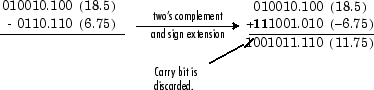`

Most fixed-point DSP System Toolbox™ blocks that perform addition cast the adder inputs to an accumulator data type before performing the addition. Therefore, no further shifting is necessary during the addition to line up the binary points. See Casts for more information.

### Multiplication

The multiplication of two's complement fixed-point numbers is directly analogous to regular decimal multiplication, with the exception that the intermediate results must be sign extended so that their left sides align before you add them together.

For example, consider the multiplication of 10.11 (-1.25) with 011 (3):

`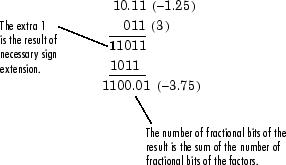`

#### Multiplication Data Types

The following diagrams show the data types used for fixed-point multiplication in the System Toolbox software. The diagrams illustrate the differences between the data types used for real-real, complex-real, and complex-complex multiplication. See individual reference pages to determine whether a particular block accepts complex fixed-point inputs.

In most cases, you can set the data types used during multiplication in the block mask. For details, see Casts.

### Note

The following diagrams show the use of fixed-point data types in multiplication in System Toolbox software. They do not represent actual subsystems used by the software to perform multiplication.

Real-Real Multiplication.  The following diagram shows the data types used in the multiplication of two real numbers in System Toolbox software. The software returns the output of this operation in the product output data type, as the next figure shows.Real-Complex Multiplication.  The following diagram shows the data types used in the multiplication of a real and a complex fixed-point number in System Toolbox software. Real-complex and complex-real multiplication are equivalent. The software returns the output of this operation in the product output data type, as the next figure shows.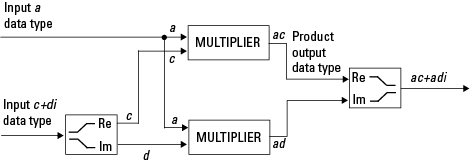Complex-Complex Multiplication.  The following diagram shows the multiplication of two complex fixed-point numbers in System Toolbox software. Note that the software returns the output of this operation in the accumulator output data type, as the next figure shows.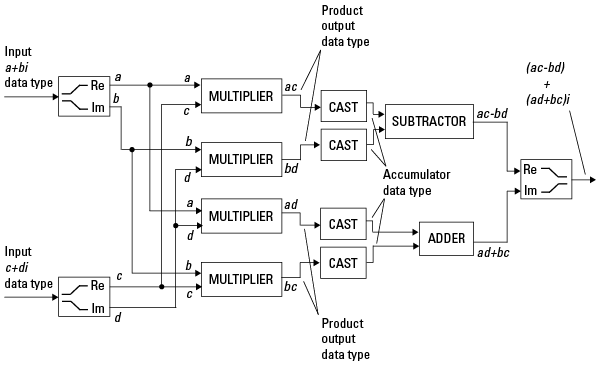System Toolbox blocks cast to the accumulator data type before performing addition or subtraction operations. In the preceding diagram, this is equivalent to the C code

```acc=ac; acc-=bd;```

for the subtractor, and

```acc=ad; acc+=bc;```

for the adder, where acc is the accumulator.

### Casts

Many fixed-point System Toolbox blocks that perform arithmetic operations allow you to specify the accumulator, intermediate product, and product output data types, as applicable, as well as the output data type of the block. This section gives an overview of the casts to these data types, so that you can tell if the data types you select will invoke sign extension, padding with zeros, rounding, and/or overflow. Sign extension is the addition of bits that have the value of the most significant bit to the high end of a two's complement number. Sign extension does not change the value of the binary number. Padding is extending the least significant bit of a binary word with one or more zeros.

#### Casts to the Accumulator Data Type

For most fixed-point System Toolbox blocks that perform addition or subtraction, the operands are first cast to an accumulator data type. Most of the time, you can specify the accumulator data type on the block mask. For details, see the description for Accumulator data type parameter in Specify Fixed-Point Attributes for Blocks. Since the addends are both cast to the same accumulator data type before they are added together, no extra shift is necessary to insure that their binary points align. The result of the addition remains in the accumulator data type, with the possibility of overflow.

#### Casts to the Intermediate Product or Product Output Data Type

For System Toolbox blocks that perform multiplication, the output of the multiplier is placed into a product output data type. Blocks that then feed the product output back into the multiplier might first cast it to an intermediate product data type. Most of the time, you can specify these data types on the block mask. For details, see the description for Intermediate Product and Product Output data type parameters in Specify Fixed-Point Attributes for Blocks.

#### Casts to the Output Data Type

Many fixed-point System Toolbox blocks allow you to specify the data type and scaling of the block output on the mask. Remember that the software does not allow mixed types on the input and output ports of its blocks. Therefore, if you would like to specify a fixed-point output data type and scaling for a System Toolbox block that supports fixed-point data types, you must feed the input port of that block with a fixed-point signal. The final cast made by a fixed-point System Toolbox block is to the output data type of the block.

Note that although you cannot mix fixed-point and floating-point signals on the input and output ports of blocks, you can have fixed-point signals with different word and fraction lengths on the ports of blocks that support fixed-point signals.

#### Casting Examples

It is important to keep in mind the ramifications of each cast when selecting these intermediate data types, as well as any other intermediate fixed-point data types that are allowed by a particular block. Depending upon the data types you select, overflow and/or rounding might occur. The following two examples demonstrate cases where overflow and rounding can occur.

Cast from a Shorter Data Type to a Longer Data Type.  Consider the cast of a nonzero number, represented by a four-bit data type with two fractional bits, to an eight-bit data type with seven fractional bits:As the diagram shows, the source bits are shifted up so that the binary point matches the destination binary point position. The highest source bit does not fit, so overflow might occur and the result can saturate or wrap. The empty bits at the low end of the destination data type are padded with either 0's or 1's:

• If overflow does not occur, the empty bits are padded with 0's.

• If wrapping occurs, the empty bits are padded with 0's.

• If saturation occurs,

• The empty bits of a positive number are padded with 1's.

• The empty bits of a negative number are padded with 0's.

You can see that even with a cast from a shorter data type to a longer data type, overflow might still occur. This can happen when the integer length of the source data type (in this case two) is longer than the integer length of the destination data type (in this case one). Similarly, rounding might be necessary even when casting from a shorter data type to a longer data type, if the destination data type and scaling has fewer fractional bits than the source.

Cast from a Longer Data Type to a Shorter Data Type.  Consider the cast of a nonzero number, represented by an eight-bit data type with seven fractional bits, to a four-bit data type with two fractional bits:As the diagram shows, the source bits are shifted down so that the binary point matches the destination binary point position. There is no value for the highest bit from the source, so the result is sign extended to fill the integer portion of the destination data type. The bottom five bits of the source do not fit into the fraction length of the destination. Therefore, precision can be lost as the result is rounded.

In this case, even though the cast is from a longer data type to a shorter data type, all the integer bits are maintained. Conversely, full precision can be maintained even if you cast to a shorter data type, as long as the fraction length of the destination data type is the same length or longer than the fraction length of the source data type. In that case, however, bits are lost from the high end of the result and overflow might occur.

The worst case occurs when both the integer length and the fraction length of the destination data type are shorter than those of the source data type and scaling. In that case, both overflow and a loss of precision can occur.

Watch now